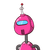# ABC are the points on circle m arc bc 110 marcAb125 find measure arc ac

ABC are the points on circle m arc bc 110 marcAb125 find measure arc ac

### 1 thought on “ABC are the points on circle m arc bc 110 marcAb125 find measure arc ac”

1.m(arc AC) = 125

Step-by-step explanation:

m(arc AB) + m(arc BC) + m(arc AC) = 360...[since the circle is 360].

125+110+ m(arc AC) = 360

235+ m(arc AC) = 360

m(arc AC) = 360-235

= 125

.’.  m(arc AC) = 125

.

.

.

HOPE IT HELPS!!!!!

HAVE A HAPPY DAY:):)